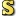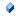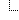﻿FVMath Class
Flexivert Library Documentation
FVMath Class
NamespacesFlexivert.UtilsFVMath
Provides basic mathematical operations commonly used in Flexivert.Declaration Syntax
 C# Visual Basic Visual C++
`public class FVMath`
`Public Class FVMath`
`public ref class FVMath`Members
 All Members Constructors Methods Fields PublicProtected InstanceStatic DeclaredInherited
IconMemberDescriptionFVMath()()()
Initializes a new instance of the FVMath classAngle(Single, Single, Single, Single)
Calculates the angle between a line and the horizontal line.Atan(Single)
Returns the angle whose tangent is the specified number.Atan2(Single, Single)
Returns the angle whose tangent is the quotient of two specified numbers.CalcControlPoint(Edge, Single, Single)
Calculates the position of the ControlPoint control point for an edge.CalcMidPoint(Edge, Single, Single)
Calculates the position of the midpoint on an edge's curve.Cos(Single)
Calculates a single-word floating-point cosine of the specified angle.Equals(Object)
Determines whether the specified Object is equal to the current Object.
(Inherited from Object.)Finalize()()()
Allows an Object to attempt to free resources and perform other cleanup operations before the Object is reclaimed by garbage collection.
(Inherited from Object.)GetHashCode()()()
Serves as a hash function for a particular type.
(Inherited from Object.)GetType()()()
Gets the Type of the current instance.
(Inherited from Object.)MemberwiseClone()()()
Creates a shallow copy of the current Object.
(Inherited from Object.)PI_F
The single-word floating point PI numberSin(Single)
Calculates a single-word floating-point sine of the specified angle.Tan(Single)
Calculates a single-word floating-point tangent of the specified angle.ToString()()()
Returns a String that represents the current Object.
(Inherited from Object.)Inheritance Hierarchy
 ObjectFVMath

Assembly: FVCore (Module: FVCore) Version: 0.5.8.1 (0.5.8.12)